## Overview

An illustration of the ADAptive LInear NEuron (Adaline) -- a single-layer artificial linear neuron with a threshold unit: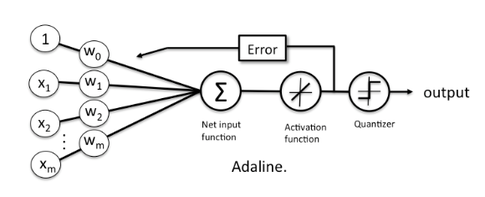The Adaline classifier is closely related to the Ordinary Least Squares (OLS) Linear Regression algorithm; in OLS regression we find the line (or hyperplane) that minimizes the vertical offsets. Or in other words, we define the best-fitting line as the line that minimizes the sum of squared errors (SSE) or mean squared error (MSE) between our target variable (y) and our predicted output over all samples $i$ in our dataset of size $n$.

LinearRegression implements a linear regression model for performing ordinary least squares regression, and in Adaline, we add a threshold function $g(\cdot)$ to convert the continuous outcome to a categorical class label:

$$y = g({z}) =$$

An Adaline model can be trained by one of the following three approaches:

• Normal Equations

### Normal Equations (closed-form solution)

The closed-form solution should be preferred for "smaller" datasets where calculating (a "costly") matrix inverse is not a concern. For very large datasets, or datasets where the inverse of $[X^T X]$ may not exist (the matrix is non-invertible or singular, e.g., in case of perfect multicollinearity), the gradient descent or stochastic gradient descent approaches are to be preferred.

The linear function (linear regression model) is defined as:

where $y$ is the response variable, $\mathbf{x}$ is an $m$-dimensional sample vector, and $\mathbf{w}$ is the weight vector (vector of coefficients). Note that $w_0$ represents the y-axis intercept of the model and therefore $x_0=1$.

Using the closed-form solution (normal equation), we compute the weights of the model as follows:

Random shuffling is implemented as:

• for one or more epochs
• randomly shuffle samples in the training set
• for training sample i

## Example 1 - Closed Form Solution

from mlxtend.data import iris_data
from mlxtend.plotting import plot_decision_regions
import matplotlib.pyplot as plt

X, y = iris_data()
X = X[:, [0, 3]] # sepal length and petal width
X = X[0:100] # class 0 and class 1
y = y[0:100] # class 0 and class 1

# standardize
X[:,0] = (X[:,0] - X[:,0].mean()) / X[:,0].std()
X[:,1] = (X[:,1] - X[:,1].mean()) / X[:,1].std()

eta=0.01,
minibatches=None,
random_seed=1)

plt.show()## Example 2 - Gradient Descent

from mlxtend.data import iris_data
from mlxtend.plotting import plot_decision_regions
import matplotlib.pyplot as plt

X, y = iris_data()
X = X[:, [0, 3]] # sepal length and petal width
X = X[0:100] # class 0 and class 1
y = y[0:100] # class 0 and class 1

# standardize
X[:,0] = (X[:,0] - X[:,0].mean()) / X[:,0].std()
X[:,1] = (X[:,1] - X[:,1].mean()) / X[:,1].std()

eta=0.01,
minibatches=1, # for Gradient Descent Learning
random_seed=1,
print_progress=3)

plt.show()

plt.xlabel('Iterations')
plt.ylabel('Cost')

Iteration: 30/30 | Cost 3.79 | Elapsed: 0:00:00 | ETA: 0:00:00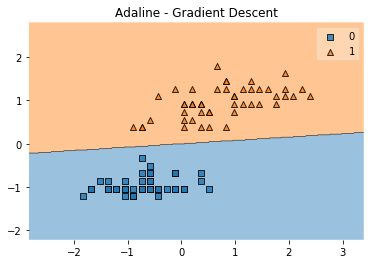Text(0, 0.5, 'Cost')## Example 3 - Stochastic Gradient Descent

from mlxtend.data import iris_data
from mlxtend.plotting import plot_decision_regions
import matplotlib.pyplot as plt

X, y = iris_data()
X = X[:, [0, 3]] # sepal length and petal width
X = X[0:100] # class 0 and class 1
y = y[0:100] # class 0 and class 1

# standardize
X[:,0] = (X[:,0] - X[:,0].mean()) / X[:,0].std()
X[:,1] = (X[:,1] - X[:,1].mean()) / X[:,1].std()

eta=0.02,
minibatches=len(y), # for SGD learning
random_seed=1,
print_progress=3)

plt.show()

plt.xlabel('Iterations')
plt.ylabel('Cost')
plt.show()

Iteration: 15/15 | Cost 3.81 | Elapsed: 0:00:00 | ETA: 0:00:00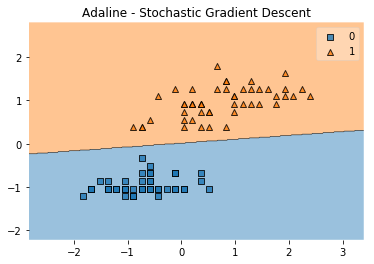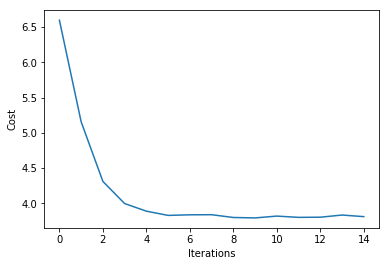## Example 4 - Stochastic Gradient Descent with Minibatches

from mlxtend.data import iris_data
from mlxtend.plotting import plot_decision_regions
import matplotlib.pyplot as plt

X, y = iris_data()
X = X[:, [0, 3]] # sepal length and petal width
X = X[0:100] # class 0 and class 1
y = y[0:100] # class 0 and class 1

# standardize
X[:,0] = (X[:,0] - X[:,0].mean()) / X[:,0].std()
X[:,1] = (X[:,1] - X[:,1].mean()) / X[:,1].std()

eta=0.02,
minibatches=5, # for SGD learning w. minibatch size 20
random_seed=1,
print_progress=3)

plt.show()

plt.xlabel('Iterations')
plt.ylabel('Cost')
plt.show()

Iteration: 15/15 | Cost 3.87 | Elapsed: 0:00:00 | ETA: 0:00:00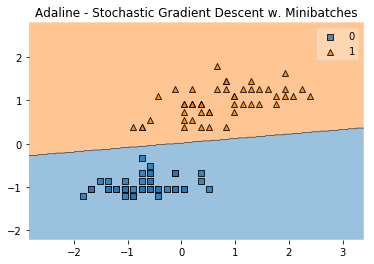# API

Note that this implementation of Adaline expects binary class labels in {0, 1}.

Parameters

• eta : float (default: 0.01)

solver rate (between 0.0 and 1.0)

• epochs : int (default: 50)

Passes over the training dataset. Prior to each epoch, the dataset is shuffled if minibatches > 1 to prevent cycles in stochastic gradient descent.

• minibatches : int (default: None)

The number of minibatches for gradient-based optimization. If None: Normal Equations (closed-form solution) If 1: Gradient Descent learning If len(y): Stochastic Gradient Descent (SGD) online learning If 1 < minibatches < len(y): SGD Minibatch learning

• random_seed : int (default: None)

Set random state for shuffling and initializing the weights.

• print_progress : int (default: 0)

Prints progress in fitting to stderr if not solver='normal equation' 0: No output 1: Epochs elapsed and cost 2: 1 plus time elapsed 3: 2 plus estimated time until completion

Attributes

• w_ : 2d-array, shape={n_features, 1}

Model weights after fitting.

• b_ : 1d-array, shape={1,}

Bias unit after fitting.

• cost_ : list

Sum of squared errors after each epoch.

Examples

### Methods

fit(X, y, init_params=True)

Learn model from training data.

Parameters

• X : {array-like, sparse matrix}, shape = [n_samples, n_features]

Training vectors, where n_samples is the number of samples and n_features is the number of features.

• y : array-like, shape = [n_samples]

Target values.

• init_params : bool (default: True)

Re-initializes model parameters prior to fitting. Set False to continue training with weights from a previous model fitting.

Returns

• self : object

get_params(deep=True)

Get parameters for this estimator.

Parameters

• deep : boolean, optional

If True, will return the parameters for this estimator and contained subobjects that are estimators.

Returns

• params : mapping of string to any

Parameter names mapped to their values.'

predict(X)

Predict targets from X.

Parameters

• X : {array-like, sparse matrix}, shape = [n_samples, n_features]

Training vectors, where n_samples is the number of samples and n_features is the number of features.

Returns

• target_values : array-like, shape = [n_samples]

Predicted target values.

score(X, y)

Compute the prediction accuracy

Parameters

• X : {array-like, sparse matrix}, shape = [n_samples, n_features]

Training vectors, where n_samples is the number of samples and n_features is the number of features.

• y : array-like, shape = [n_samples]

Target values (true class labels).

Returns

• acc : float

The prediction accuracy as a float between 0.0 and 1.0 (perfect score).

set_params(params)

Set the parameters of this estimator. The method works on simple estimators as well as on nested objects (such as pipelines). The latter have parameters of the form <component>__<parameter> so that it's possible to update each component of a nested object.

Returns

self